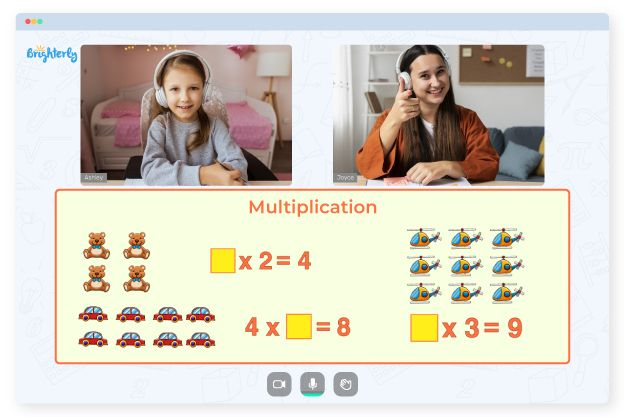One of the essential math concepts kids learn is multiplication. Understanding of multiplication is the foundation for the development of resource management, scaling, and expansion skills. Grade 3 multiplication worksheets help kids understand what expansion is all about and expose that knowledge to various forms of multiplication like missing factors, word problems, and multiple-digit multiplication.

## Free 3rd Grade Multiplication Worksheets from Brighterly’s Tutors

Brighterly’s free multiplication worksheets grade 3 are aimed at helping kids learn the concept of multiplication and memorizing the times table using exercises. These free multiplication worksheets for the 3rd grade include exercises on column multiplication and missing factor questions.

For kids in the 3rd grade, multiplication may be a complex concept. Therefore, it takes lots of repetition to grasp new information. With Brighterly’s free multiplication sheets for 3rd graders, you can ensure your kids understand how to solve problems and apply multiplication concepts in the real world.

Times tables and column multiplication are essential components of mathematics. When kids understand them, they will solve multiplication problems faster. Furthermore, basic multiplication problems like multiplying two by one are the first stage of mastering column multiplication, and it begins in the 3rd grade.

With free 3rd grade multiplication worksheets from Brighterly, your kids will get the best materials with introduction to multiplication. They will also learn to enjoy the process, and whenever they meet a steep learning curve, you can step in and help them progress.

Math for Kids

Is Your Child Struggling With Math?
1:1 Online Math Tutoring## Printable PDFfs of Grade 3 Multiplication Worksheets

Many 3rd graders find math complex and tedious because they don’t have materials they can use for review after school lessons. But there is a solution. You can help your kids understand multiplication by downloading Brighterly’s printable multiplication worksheets for grade 3 in PDF files and practicing with them.### Multiplication with Pictures### Multiplication Tables### Multiplication Word Problems## Good Times Await Students with Multiplication Worksheets for Grade 3

Practicing with printable multiplication worksheets for grade 3, students can learn strategies that are useful for their development. These worksheets make kids use a step-by-step method to solve math problems, which is essential in boosting their problem-solving abilities.

Images in the 3rd grade math worksheets are essential in helping kids visualize and simplify complex concepts. Well-designed multiplication worksheets for grade 3 students are among the best investments you can make in your child’s education.

### More Multiplication Worksheets

Problems with Multiplication?• Is your child finding multiplication challenging to grasp?
• An online tutor could be the solution.

Does your child struggle to understand multiplication concepts? Start lessons with an online tutor.